# 带你快速掌握PHP数组元素的替换（实例详解）`array_splice`函数

`array_splice(array,start,length,array)`

```<?php
\$a1=array("a"=>"red","b"=>"green","c"=>"blue","d"=>"yellow");
\$a2=array("a"=>"purple","b"=>"orange");
array_splice(\$a1,0,2,\$a2);
print_r(\$a1);
?>```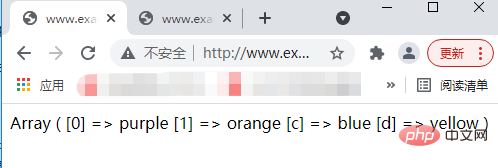`array_replace`函数

`array_replace(array1,array2,array3...)`

```<?php
\$a1=array("a"=>"red","b"=>"green");
\$a2=array("a"=>"orange","burgundy");
print_r(array_replace(\$a1,\$a2));
?>```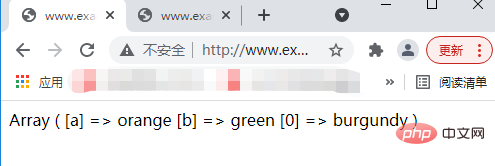```<?php
\$a1=array("a"=>"red","green");
\$a2=array("a"=>"orange","b"=>"burgundy");
print_r(array_replace(\$a1,\$a2));
?>```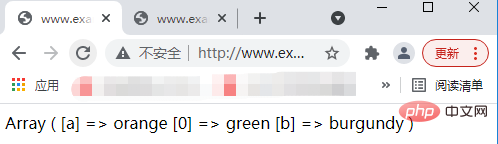```<?php
\$a1=array("red","green");
\$a2=array("blue","yellow");
\$a3=array("orange","burgundy");
print_r(array_replace(\$a1,\$a2,\$a3));
?>```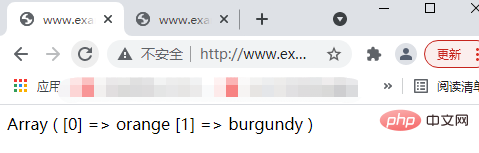`array_replace_recursive`函数

`array_replace_recursive`函数的基本语法格式如下：

`array_replace_recursive(array1,array2,array3...)`

```<?php
\$a1=array("a"=>array("red"),"b"=>array("green","blue"));
\$a2=array("a"=>array("yellow"),"b"=>array("black"));
\$a3=array("a"=>array("orange"),"b"=>array("burgundy"));
print_r(array_replace_recursive(\$a1,\$a2,\$a3));
?>```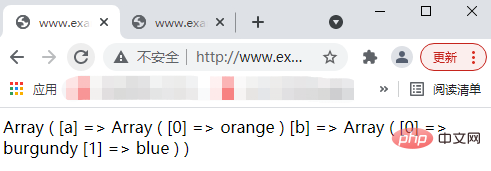1/1